# Fast, Secure 2-of-2 ECDSA using DKLs18

By Author

Engineering

, November 1, 2021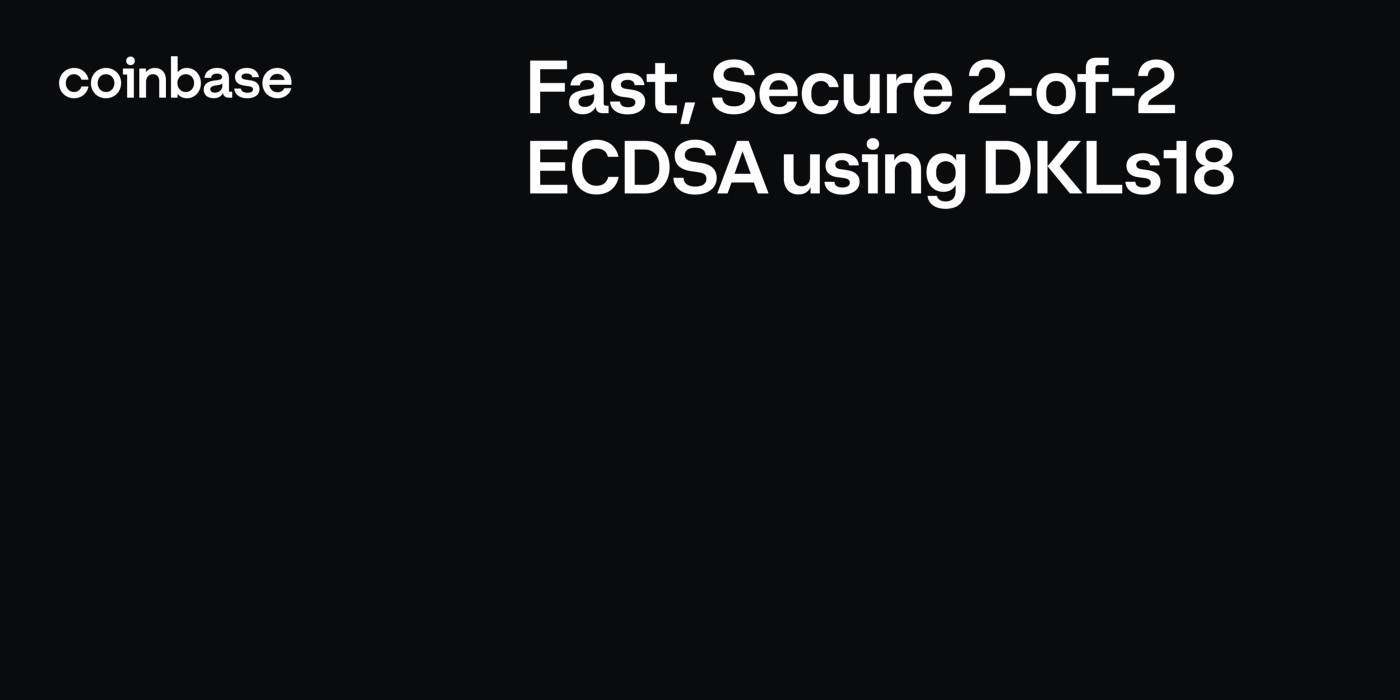We’ve already discussed the general notions of digital signatures and threshold signatures in another post. At Coinbase, we’ve focused our attention specifically on two-party threshold signing; we’ll discuss this setting in this post.

DKLs: an introduction. While threshold-signing protocols for the Schnorr and BLS schemes are relatively straightforward, threshold ECDSA is much harder. A number of protocols exist for 2-of-2 ECDSA signing; some target it explicitly (i.e., they don’t support more general choices of t and n). In this purely expository blog post, we’ll study one in particular: the 2018 protocol of Doerner, Kondi, Lee and shelat. This protocol builds on prior work of Chou and Orlandi and Keller, Orsini and Scholl. This protocol — or “DKLs”, for short — allows two parties to securely generate an ECDSA signature in just a handful of milliseconds, by exchanging just 3 messages, and all the while transmitting about 120 total kilobytes of data. In the process, it uses a few interesting techniques in secure two-party computation, and represents a disciplined, striking contribution to this field.

Let’s now say a few technical things about how DKLs works. The basic idea has to do with multiplicative secret-sharing of prime-field elements. If our two parties — Alice and Bob, say — locally generate random scalars sk_A and sk_B in 𝔽_q, then, after performing a Diffie–Hellman-like exchange, the parties may mutually derive the jointly owned public key P = sk_A · sk_B · G, without learning anything about each other’s respective key-shares (or the joint secret key). The joint secret key is the product sk := sk_A · sk_B (mod q). DKLs is unusual in its use of multiplicative sharing; the protocol fails to generalize to the (t, n) setting for essentially this reason.

The parties begin the process of ECDSA-signing a particular message m in an analogous way. They generate individual nonce-shares k_A and k_B randomly, and construct R := k_A · k_B · G as they did P; using R’s coordinates (x, y), they both acquire the first signature component r := x (mod p). It remains only for the parties to jointly construct s := H(m) · k⁻¹ + r · sk · k⁻¹ (mod q), as prescribed by the definition of ECDSA. The trouble is that the parties only possess multiplicative sharings of the quantities k and sk, and not the required values themselves.

DKLs observes that the expression defining s can just as well be written as: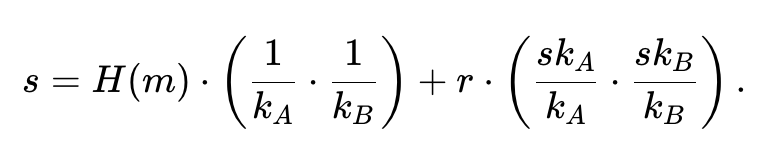The first key idea is that it would be enough for the parties to obtain random additive sharings of the two product expressions 1/k_A · 1/k_B and sk_A / k_A · sk_B / k_B above. Indeed, if the parties were to obtain such sharings, then they could both proceed by multiplying these local shares by H(m) and r, respectively, and adding the results (recall that both parties know H(m) and r). Upon doing so, the parties would acquire additive sharings of s itself, which they could finally safely exchange (i.e., without leaking any further information about s’s individual summands). Note finally that Alice can locally compute the left-hand multiplicands 1/k_A and sk_A/k_A; Bob can likewise locally compute the right-hand multiplicands 1/k_B and sk_B/k_B.

It’s thus enough to handle the problem of “secure multiplication”, also sometimes termed “multiplicative-to-additive conversion”. In this problem, two parties submit respective input scalars i_A and i_A, and wind up with random additive shares t_a and t_b of the product i_A · i_B (mod q). In other words, the identity t_A + t_B = i_A · i_B (mod q) should hold, and moreover the outputs t_A and t_B should be random subject to this condition; finally, the parties must learn nothing about each other’s inputs i_A and i_B in the process of executing the protocol (even if they engage in malicious behavior).

DKLs describes a fascinating secure multiplication subprotocol, using a further primitive called correlated oblivious transfer (cOT for short). In a cOT protocol, the parties Alice and Bob have asymmetric roles. Alice inputs a single scalar, say ɑ, in 𝔽_q; Bob inputs just a single bit b. The parties each receive a scalar as output; let’s again call these t_A and t_B for now. By definition of cOT, the outputs t_A and t_B should be random subject to the condition that t_A + t_B = ɑ if Bob’s input bit b is 1 and random subject to t_A + t_B = 0 if Bob’s input bit is 0. Either way, the sender should learn nothing about the receiver’s bit b, and the receiver should learn nothing about the sender’s scalar ɑ. The definition of correlated oblivious transfer is illustrated in the figure below.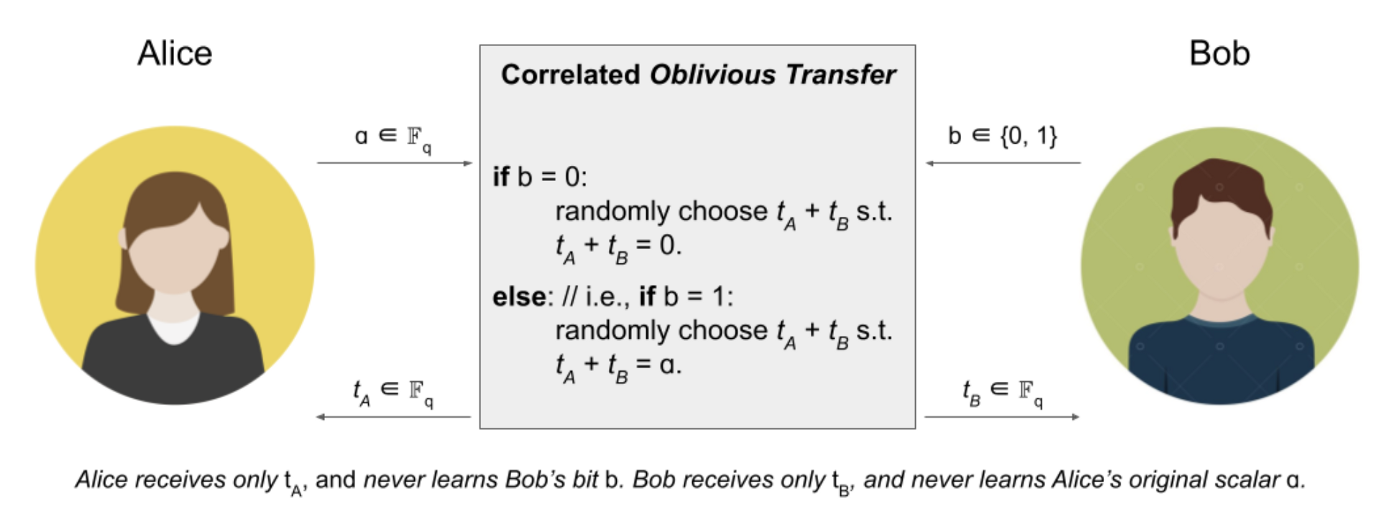Assuming that we have a cOT protocol in hand, how can we bootstrap it into a multiplication protocol? Interestingly, Alice and Bob can use an algorithm from elementary school to do this. Let’s recall the so-called long multiplication algorithm. Roughly, the procedure successively shifts the top multiplicand to the left by one place at a time; in each step, it also multiplies this multiplicand by the appropriate digit of the lower multiplicand. Finally, it adds the resulting array of shifted and multiplied numbers. In binary, things become even simpler, because the lower multiplicand’s “digits” are each either 0 or 1. A figure depicting this process is shown below: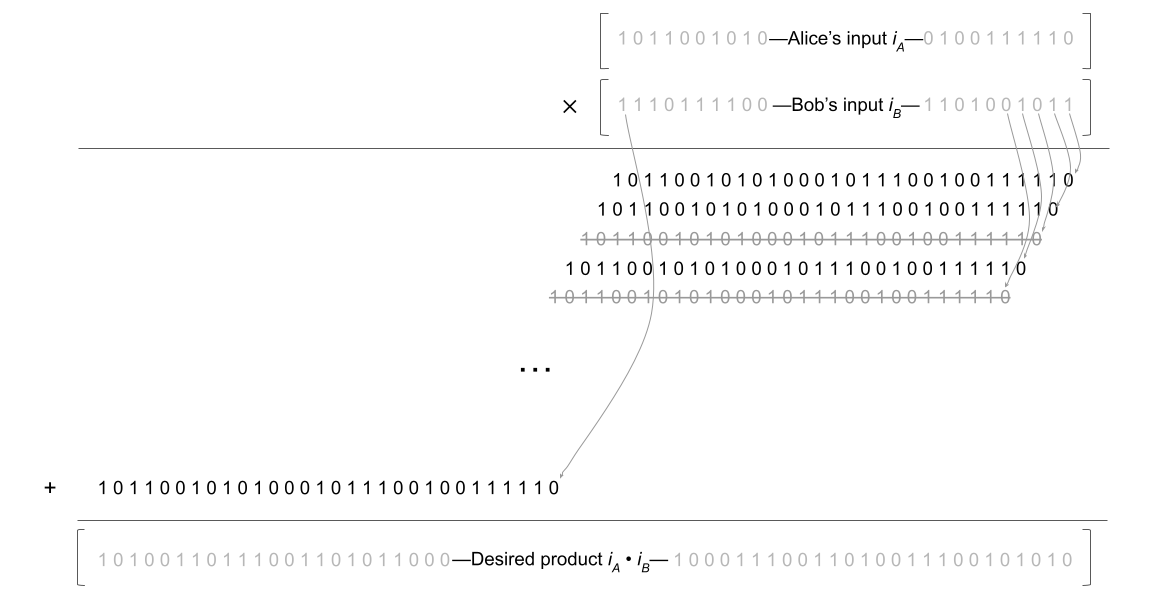In each row of this figure, Alice’s original input is shifted a further step to the left. Furthermore, working right-to-left through Bob’s input, we also strike out the rows corresponding to the bits where Bob’s input is 0. Finally, we add up the resulting numbers to obtain the product. (In reality, everything here happens mod q, but let’s ignore that for clarity; we’ve also simplified various aspects of the multiplication protocol for expository purposes.)

The insight here is that we can use cOT to do this securely. Indeed, each row of the above diagonal array can be handled by exactly one correlated oblivious transfer. Alice inputs her original input i_A, appropriately shifted by j steps to the left; Bob inputs the jᵗʰ bit of his original input i_B. By definition of cOT, the parties end up with random additive shares modulo q of either 0 or 2ʲ · i_A, depending on Bob’s bit. By doing this for each row individually, the parties obtain additive sharings of each row above, while learning nothing about each other’s inputs in the process. Finally, by adding the local additive shares so obtained, the parties wind up with additive shares of the entire product i_A · i_B. This is exactly what we wanted above.

Future work. The techniques of DKLs are powerful and interesting; this makes its techniques easier to understand, generalize, and possibly improve. The main drawback of DKLs is its bandwidth requirement, which stands at a relatively high ~120KB (total bytes exchanged). It would be intriguing to try to lessen this bandwidth requirement, by improving DKLs’s techniques. We’ve considered trying to improve this bandwidth using a Karatsuba-like approach, but haven’t managed to put together the details yet. Roughly, Karatsuba works by cleverly replacing one product of full-length numbers by three products of half-length numbers (and handling these products recursively, and so on). The key fact which makes this approach faster is that multiplying two half-length numbers takes only a quarter of the work as multiplying two full-length numbers (because of the quadratic amount of work involved; see above). All said, Karatsuba can multiply two n-bit numbers in just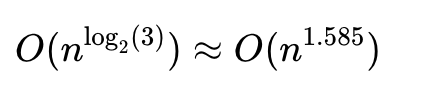atomic operations, as opposed to the O(n²) required by naïve multiplication. The problem with applying this technique to DKLs is that the outputs of each cOT need to be random modulo q. This forces each output to take up log q bits, even when the actual numbers involved are actually significantly smaller than q. This nullifies the benefits which Karatsuba is supposed to convey — because halving the length no longer correspondingly halves the bandwidth. It’s possible that this could be made to work using a few new ideas.

* * *

If you are interested in cutting-edge cryptography, check out our open roles here.

## Take control of your money. Start your portfolio today and get crypto.

Sign up for a Coinbase account today and see what the world of decentralized finance can do for you.